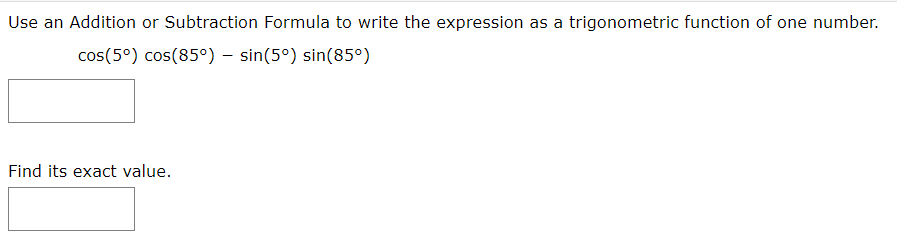# Use an Addition or Subtraction Formula to write the expression as a trigonometric function of one number.cos(5°) cos(85°) - sin(5°) sin(85°)Find its exact value.

Question
2 viewshelp_outlineImage TranscriptioncloseUse an Addition or Subtraction Formula to write the expression as a trigonometric function of one number. cos(5°) cos(85°) - sin(5°) sin(85°) Find its exact value. fullscreen
check_circle

Step 1

cosA*cosB-sinA*sinB= cos(A+B)

Here A=5 and...

### Want to see the full answer?

See Solution

#### Want to see this answer and more?

Solutions are written by subject experts who are available 24/7. Questions are typically answered within 1 hour.*

See Solution
*Response times may vary by subject and question.
Tagged in